# Maharashtra Board Practice Set 12 Class 7 Maths Solutions Chapter 3 HCF and LCM

Balbharti Maharashtra State Board Class 7 Maths Solutions covers the 7th Std Maths Practice Set 12 Answers Solutions Chapter 3 HCF and LCM.

## HCF and LCM Class 7 Practice Set 12 Answers Solutions Chapter 3

Question 1.
i. 25, 40
ii. 56, 32
iii. 40, 60, 75
iv. 16, 27
v. 18, 32,48
vi. 105, 154
vii. 42, 45, 48
viii. 57, 75, 102
ix. 56, 57
x. 777, 315, 588
Solution:
i. 25, 40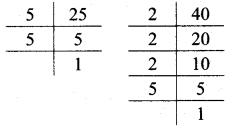∴ 25 = 5 × 5
40 = 2 × 2 × 2 × 5
∴ HCF of 25 and 40 = 5

ii. 56, 32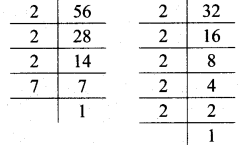∴ 56 = 2 × 2 × 2 × 7
32 = 2 × 2 × 2 × 2 × 2
∴ HCF of 56 and 32 = 2 × 2 × 2
∴ HCF of 56 and 32 = 8

iii. 40, 60, 75∴ 40 = 2 × 2 × 2 × 5
60 = 2 × 2 × 3 × 5
75 = 3 × 5 × 5
∴ HCF of 40, 60 and 75 = 5

iv. 16, 27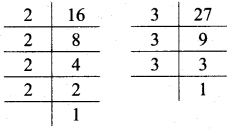∴ 16 = 2 × 2 × 2 × 2 × 1
27 = 3 × 3 × 3 × 1
∴ HCF of 16 and 27 = 1

v. 18, 32,48∴ 18 = 2 × 3 × 3
32 = 2 × 2 × 2 × 2 × 2
48 = 2 × 2 × 2 × 2 × 3
∴ HCF of 18, 32 and 48 = 2

vi. 105, 154∴ 105 = 3 × 5 × 7
154 = 2 × 2 × 11
∴ HCF of 105 and 154 = 7

vii. 42, 45, 48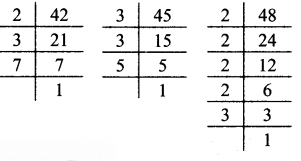∴ 42 = 2 × 3 × 7
45 = 3 × 3 × 5
48 = 2 × 2 × 2 × 2 × 3
∴ HCF of 42,45 and 48 =3

viii. 57, 75, 102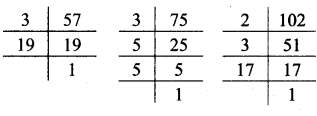∴ 57 = 3 × 19
75 = 3 × 5 × 5
102 = 2 × 3 × 17
∴ HCF of 57, 75 and 102 = 3

ix. 56, 57∴ 56 = 2 × 2 × 2 × 7 × 1
57 = 3 × 19 × 1
∴ HCF of 56 and 57 = 1

x. 777, 315, 588∴ 777 = 3 × 7 × 37
315 = 3 × 3 × 5 × 7
588 = 2 × 2 × 3 × 7 × 7
∴ HCF of 777, 315 and 588 = 3 × 7
HCF of 777, 315 and 588 = 21

Question 2.
Find the HCF by the division method and reduce to the simplest form:
i. $$\frac { 275 }{ 525 }$$
ii. $$\frac { 76 }{ 133 }$$
iii. $$\frac { 161 }{ 69 }$$
Solution:
i. $$\frac { 275 }{ 525 }$$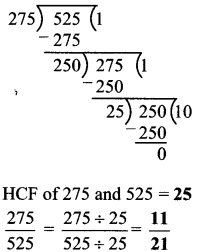ii. $$\frac { 76 }{ 133 }$$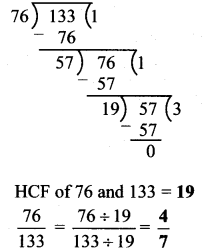iii. $$\frac { 161 }{ 69 }$$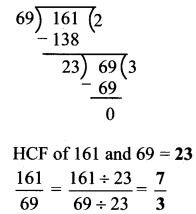Maharashtra Board Class 7 Maths Chapter 3 HCF and LCM Practice Set 12 Intext Questions and Activities

Question 1.
In each of the following examples, write all the factors of the numbers and find the greatest common divisor. (Textbook pg. no. 17)
i. 28, 42
ii. 51, 27
iii. 25, 15, 35
Solution:
i. Factors of 28 = 1,2,4, 7, 14, 28
Factors of 42 = 1,2, 3, 6, 7, 14, 21, 42
∴ HCF of 28 and 42 = 14

ii. Factors of 51 = 1, 3, 17, 51
Factors of 27 = 1, 3, 9, 27
∴ HCF of 51 and 27 = 3

iii. Factors of 25 = 1, 5, 25
Factors of 15 = 1, 3, 5, 15
Factors of 35 = 1, 5, 7, 35
∴ HCF of 25, 15 and 35 = 5

Scroll to Top